# How to Program Your Way Out of a Paper Bag Using Genetic Algorithms

How to Program Your Way Out of a Paper Bag Using Genetic Algorithms

By Frances Buontempo

It is often claimed people cannot program their way out of a paper bag. Frances Buontempo bucks the trend using genetic algorithms.

It is frequently suggested that people cannot program their way out of a paper bag, and a quick Google for the state-of-the-art currently reveals nothing until FizzBuzz is added to the search terms. This adds weight to the claim, suggesting people implement FizzBuzz rather than program their way out of a paper bag. I would therefore like to demonstrate one approach to programming one’s way out of a paper bag using genetic algorithms. Genetic algorithms, GA, are a form of evolutionary algorithm, drawing on ideas from natural evolution to find solutions to problems. John Holland brought GA to attention with his book, Adaptation in Natural and Artificial Systems . They can be used to solve a variety of problems, indeed Holland [ Holland92 ] says

Computer programs that ‘evolve’ in ways that resemble natural selection can solve complex problems even their creators do not fully understand

## Problem statement

Since the requirements of our current problem are a little vague, this implementation will assume a rectangular, unmoving paper bag of any colour. A projectile will be fired from an imaginary cannon at the bottom centre of the bag at a velocity and angle of elevation to be decided. A projectile is considered ‘out’ of a paper bag when it has fired over the top of the bag thereby disallowing bursting through the sides or the bottom. If it hits the side or bottom of the bag it will stick there. These decisions are arbitrary and any different assumptions are equally valid and would lead to different solutions. It is possible to solve the problem without an imaginary cannon, but we will leave that for another article.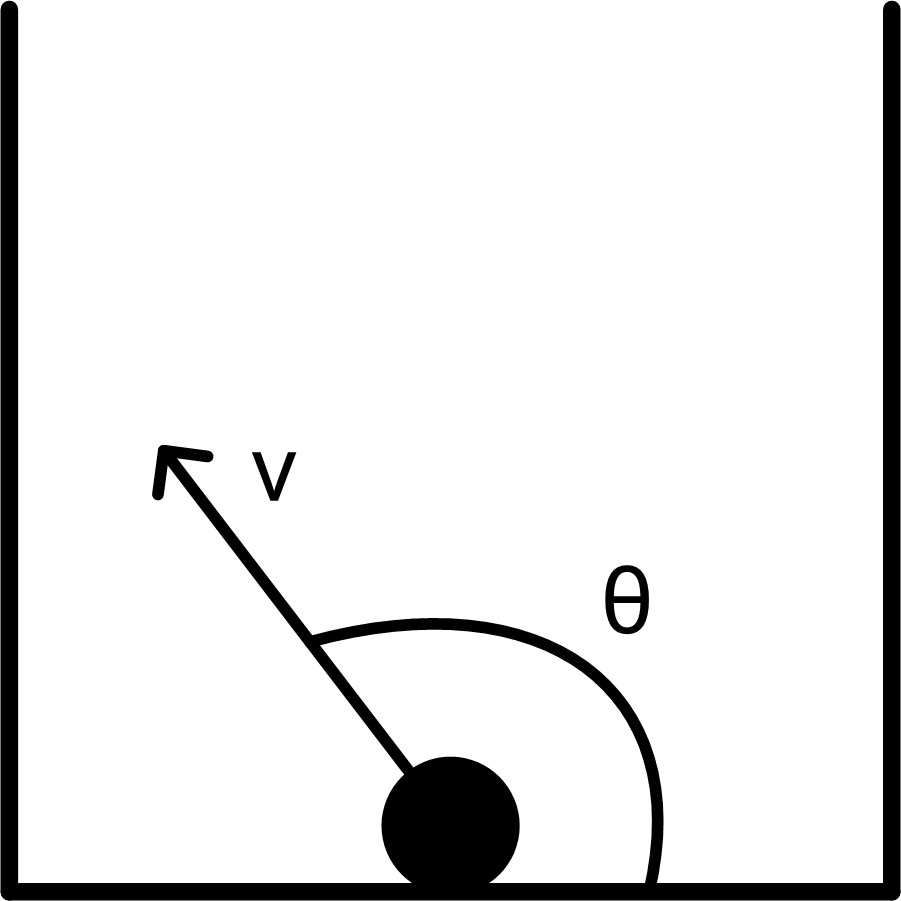Figure 1

Clearly, the problem has a closed-form range of solutions for possible angles and required velocities, but this article will show how to discover the solutions programmatically.

Given a bag with bottom left corner at ( k , 0), of width w , and height h , assuming the projectile is smaller than the bag, the cannon is a point of no size, and given the acceleration due to gravity, g , after time t the projectile will be at point ( x , y ) where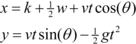Here x is the horizontal displacement and y the vertical displacement. The projectile will just escape when y h and x < k or x > k + w . Clearly as the projectile moves further left or right the height y can be below the height of the bag, h , even if the height was greater than h at the edges since the projectile will start to come down again at some point. g will be taken as 9.81 m/s2. For simplicity, the code will assume k is zero.

## Problem solution

A range of solutions can be found using genetic algorithms, along with other machine learning techniques. Initially, we form a generation of projectiles of some pre-specified size, say n , with random angles, θ , and velocities, v , and fire these from the cannon to see where they end up. We then form a new generation by randomly selecting two of the better parents from the last generation, performing crossover, wherein a child has a v ‘gene’ from one parent and a θ ‘gene’ from the other. ‘Better’ will need to be quantified, though at this stage intuitively a projectile that escapes the paper bag is better than one which does not. If two projectiles fail to escape, we must provide a criterion for choosing the better, though initially we decide they are both unacceptable to simplify things. Pairs of parents breed in this fashion until the next generation also has n items. The genes (angle and velocity) of some randomly selected children may then be mutated, wherein the values are changed slightly. This ensure the generations do not get stuck in the same place and explore more of the solution space. The generations breed for a pre-specified number of steps.

1. Create n ballistics as pairs of random ( v , θ )
2. Fire the n ballistics and note which escape
3. For each epoch
1. Make n new ballistics by:

If no ballistics escape randomly, create a new pair

Else randomly choose two parents that escaped, and

• Cross-over: take v from one and θ from the other to create a new ballistic
• Mutate: Possibly randomly change either number by a small amount
2. Kill off the last generation and repeat with the new gene pool

## Implementation in Python

Full details can be found in the code available on github [ Github ]. The main function, given in Listing 1, runs the pseudo code just presented. The ``` display_results ``` function uses MatPlotLib to create the graphs given in the results section.

 ```if __name__ == "__main__": epochs = 10 items = 12 height = 5 width = 10 generation = init_random_generation(items) generation0 = list(generation) results = launch(generation, height, width) results0 = list(results) for i in range(1, epochs): generation = crossover(generation, results, height, width) mutate(generation) results = launch(generation, height, width) display_results(generation0, results0, generation, results, height, width) ``` Listing 1

The initial generation is simply formed by randomly populating a list of angle and velocity tuples as shown in listing 2. The launch function then loops from a time, t , of 0 to a hard-coded value of 20 in steps of 0.2, finding the values of x and y for each t from the equations modelling the path of the projectile given earlier. It uses linear interpolation between each time step to decide if the projectile has hit the side of the bag, and makes it stick if it does. For brevity, it is not listed here.

 ```def init_random_generation(items): generation = [] for i in range(items): theta = random.uniform(15, 180) * math.pi/180 v = random.uniform(2, 20) generation.append((theta, v)) return generation ``` Listing 2

The crossover operation chooses two parents from any projectiles in the previous generation which escaped, as shown in listing 3. If none escaped, then a new random item is created. This may prove to be a foolish decision. This is called in a loop until the next generation is complete.

 ```def get_choices(generation, height, width, results): return [(generation[i], generation[i]) \ for i in range(len(generation)) if escaped(height, width, results[i]) ] def crossover(generation, results, height, width): choices = get_choices(generation, height, width, results) if len(choices) == 0: return init_random_generation(items) next_generation = [] for i in range(0, len(generation)): mum = generation [random.randint(0, len(choices)-1)] dad = generation [random.randint(0, len(choices)-1)] t = (mum, dad) next_generation.append(t) return next_generation ``` Listing 3

## Results

Results presented here use the parameters epochs = 10, items = 5, height = 5 and width = 10. Initially one projectile escaped, and on the tenth epoch none escaped (see Figure 2). In this instance, few escape initially, which doesn’t give enough chance for children forming the next generation to learn, since we have gone for an all or nothing strategy, just choosing projectiles that escape as suitable parents and resorting to new random ones if none escape.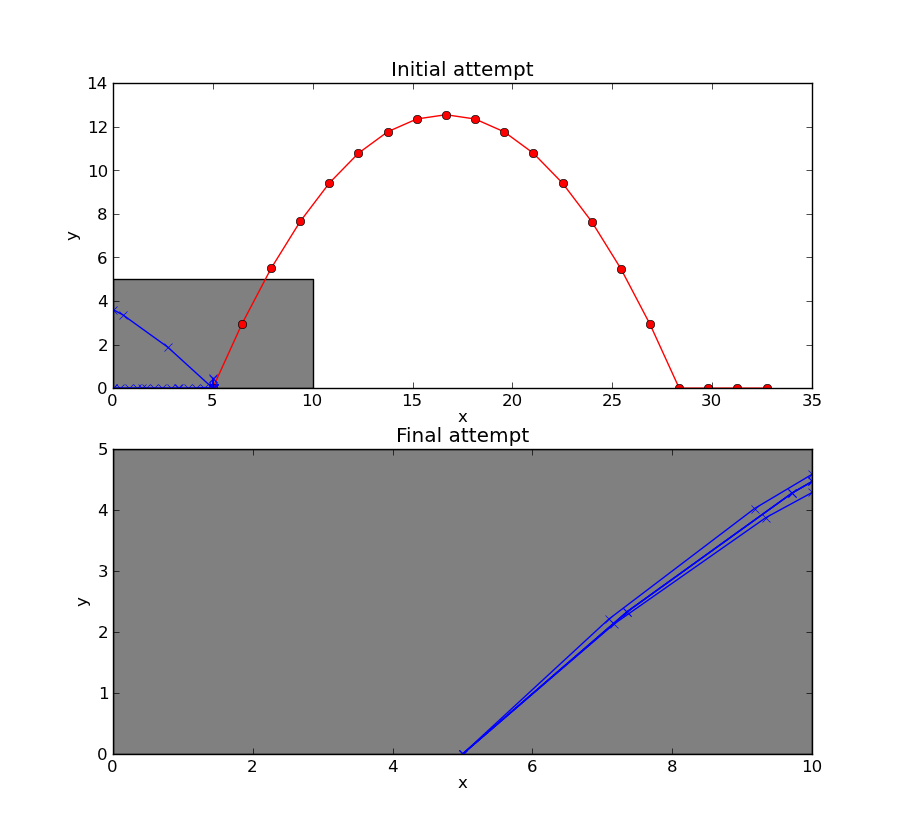Figure 2

Leaving all parameters the same, but increasing items to 25, better results are obtained. Initially six projectiles escaped, and on the tenth epoch eight escaped (see Figure 3). This improvement may come about because more initial projectiles happened to escape thereby providing more suitable parents. Initial investigations indicate using at least 12 items for the given bag size and number of epochs do well enough.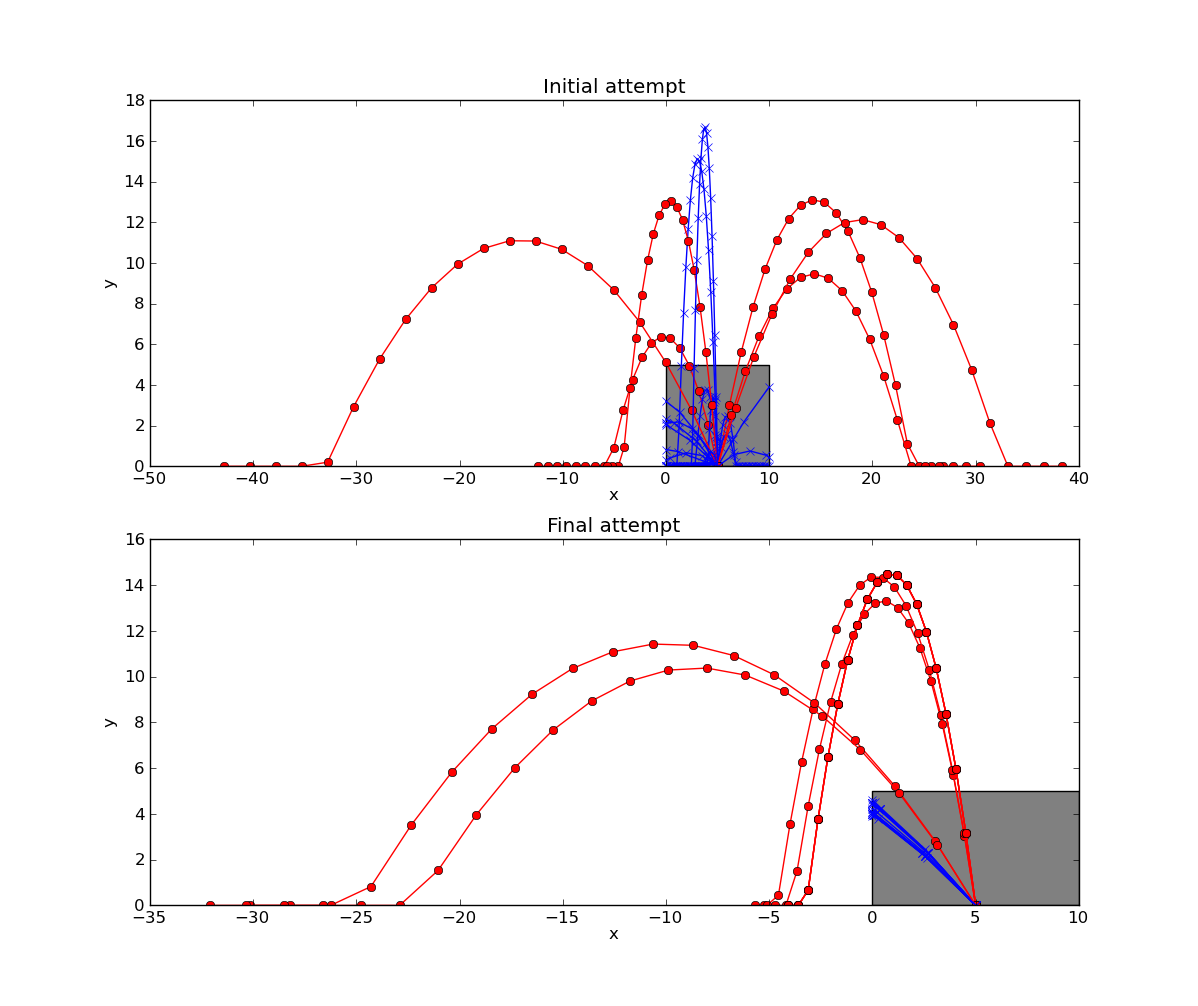Figure 3

The crossover function will chose parents from projectiles that escaped. What should be done if no projectiles escaped? In version 1, a new random generation is created. This is wasteful since even when none escape some will still do better than others, for a suitable definition of better, so this approach will lose some of the information the algorithm has discovered. We require a scoring system, known as a fitness function, to decide which pairs are better than others.

## Step back and think

We can directly find the height, y , when the x position is at the edge of the bag, rather than the approach taken in phase 1 where interpolation was used at the point just before and then just after the edge of the bag to decide if the height was great enough to escape. This will allow us to ascribe a score for each choice of angle and velocity without looping through each time-step and allow us to use the parabolic equation, rather than approximating with linear interpolation. Furthermore Figure 4 shows what can happen when the ballistic just sneaks over the bag. If we interpolate using a straight line between two positions at adjacent time points, the ballistic will appear to hit the edge of the bag, when it in fact would miss.

 Linear interpolation of a curve may lead to incorrect decisions about whether a projectile has gone over the top of the paper bag or broken through the side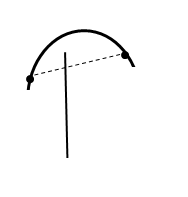Figure 4

The main difference from the first approach is a change to the parent selection. The height where the projectile crosses the bag is used as the fitness score of the gene. This means projectiles which get higher are more likely to be chosen as parents, increasing the chance of an item escaping, and using information from items which do not escape, allowing the next generation to learn from the mistakes of its parents. The new approach is shown in Listing 4.

 ```def cumulative_probabilities(results): cp = [] total = 0 for res in results: total += res cp.append(total) return cp def get_choices(generation, height, width, results): choices = cumulative_probabilities(results) return choices def choose(choices): p = random.uniform(0,choices[-1]) for i in range(len(choices)): if choices[i] >= p: return i return i def crossover(generation, results, height, width): choices = get_choices(generation, height, width, results) next_generation = [] for i in range(0, len(generation)): mum = generation[choose(choices)] dad = generation[choose(choices)] t = (mum, dad) next_generation.append(t) return next_generation ``` Listing 4

## Further results

The results in this section are for 12 items, keeping the bag width and height and number of epochs at the values stated in attempt 1. The first run is a complete success, as shown in Figure 5. With just one initial escape, all of the final generation have been programmed out of the paper bag, in stark contrast with the first approach.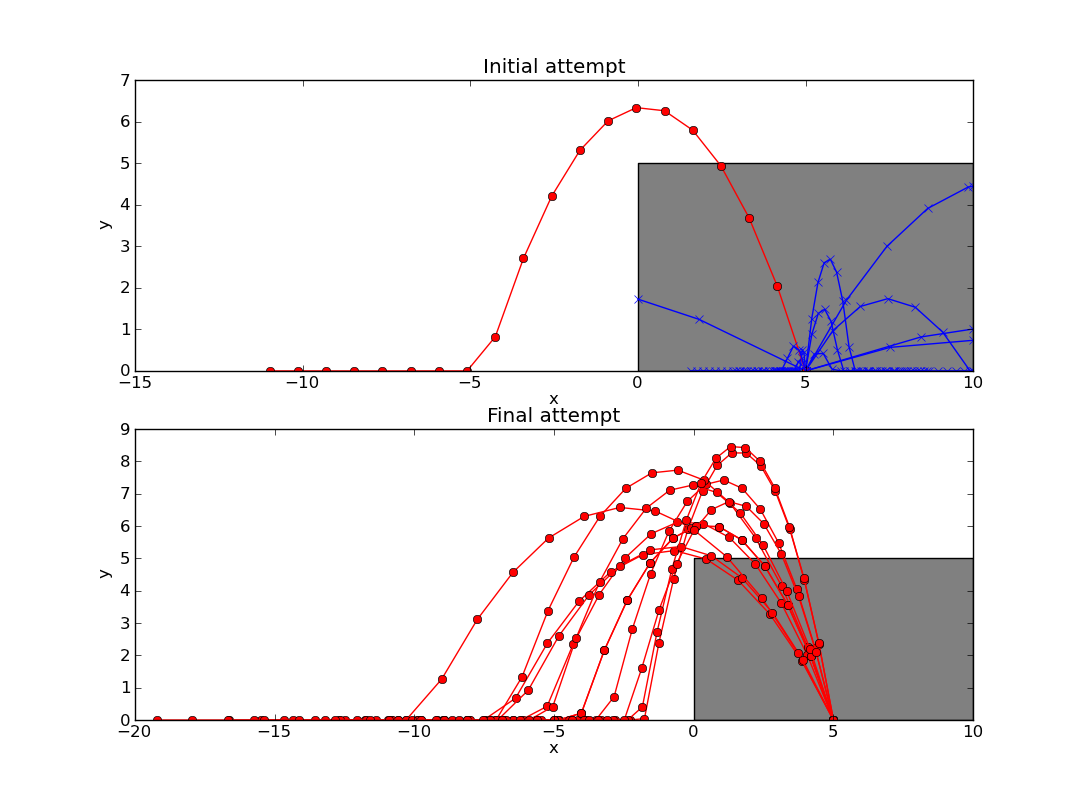Figure 5

The second run, Figure 6, demonstrates that even if no projectiles escape initially the algorithm still finds successfully genes. It can use parameters from the better projectiles, i.e. those which got higher up the bag if they hit the edge of the bag, allowing them to escape in future generations.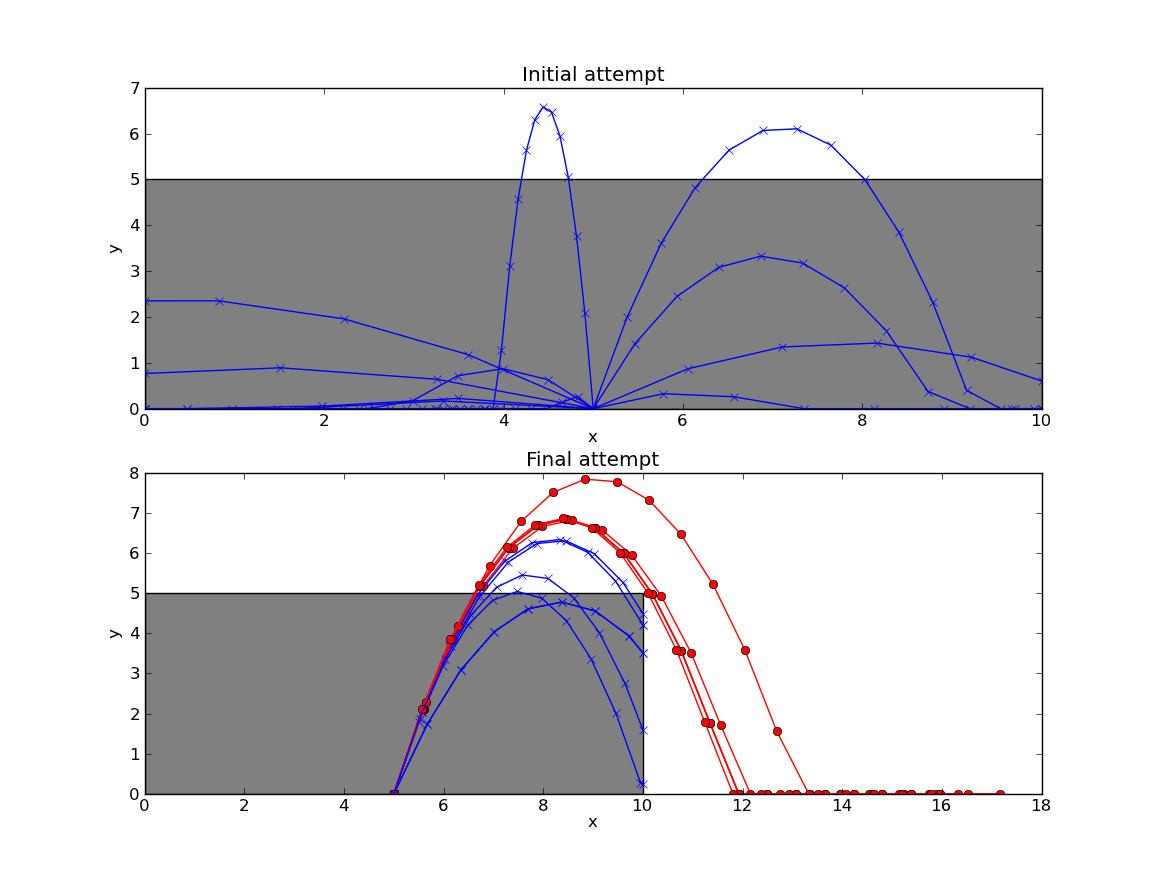Figure 6

## Conclusion

Using the idea of programming one’s way out of a paper bag can provide a variety of ways to learn a language and a new algorithm. This article has demonstrated how to use genetic algorithms to escape from a paper bag. The results presented could do with more rigorous analysis, for example to demonstrate an improvement between the techniques, the results of several runs should be compared. Ultimately, discovering a range of solutions for possible angles and required velocities or finding parameters which allow all the projectiles to escape from the paper bag would fully solve the problem. We could also consider what happens on other planets, specifically varying the value of gravity. The purpose of this article has been to demonstrate one over-engineered approach to programming one’s way out of a paper bag. There are, of course, many possible simpler approaches to the problem, but it is hoped this will serve as a gentle introduction to genetic algorithms and will inspire others to try to programme their way out of a paper bag.

## References

[Holland92] Scientific American , Vol. 267 (1992), pp. 66–72